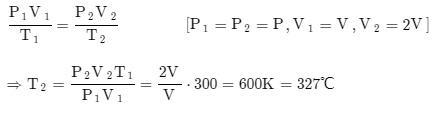Courses

# Test: Thermodynamics Level - 2

## 25 Questions MCQ Test Mechanical Engineering SSC JE (Technical) | Test: Thermodynamics Level - 2

Description
This mock test of Test: Thermodynamics Level - 2 for Mechanical Engineering helps you for every Mechanical Engineering entrance exam. This contains 25 Multiple Choice Questions for Mechanical Engineering Test: Thermodynamics Level - 2 (mcq) to study with solutions a complete question bank. The solved questions answers in this Test: Thermodynamics Level - 2 quiz give you a good mix of easy questions and tough questions. Mechanical Engineering students definitely take this Test: Thermodynamics Level - 2 exercise for a better result in the exam. You can find other Test: Thermodynamics Level - 2 extra questions, long questions & short questions for Mechanical Engineering on EduRev as well by searching above.
QUESTION: 1

Solution:
QUESTION: 2

Solution:
QUESTION: 3

### Which of the following quantities is not the property of the system

Solution:
QUESTION: 4

According to avogadro's law, for a given pressure and temperature, each molecule of a gas

Solution:
QUESTION: 5

Mixture of ice and water form a

Solution:
QUESTION: 6

Which of the following is the property of a system

Solution:
QUESTION: 7

On weight basis, air contains following parts of oxygen

Solution:
QUESTION: 8

Which of the following is not the intensive property

Solution:
QUESTION: 9

Which of the following items is not a path function

Solution:
QUESTION: 10

Work done in an adiabatic process between a given pair of end state depends on

Solution:
QUESTION: 11

Heat and work are

Solution:
QUESTION: 12

Which of the following parameters is constant for a mole for most of the gases at a given temperature and pressure

Solution:
QUESTION: 13

Which of the following quantities do not represent the property of the system.

Solution:
QUESTION: 14

Which is true for reversible polytropic process

Solution:
QUESTION: 15

If the value of n is high in the polytropic process, then the compressor work between given pressure limits will be

Solution:
QUESTION: 16

Solid and liquids have

Solution:
QUESTION: 17

A perfect gas at 27°C is heated at constant pressure till its volume is double. The final temperature is

Solution:QUESTION: 18

Work done is zero for the following process

Solution:
QUESTION: 19

One volume basis, air contains following parts of oxygen

Solution:
QUESTION: 20

The molecular weight expressed in gm (i.e., 1 gm mole) of all gases at N.T.P. occupies a volume of

Solution:
QUESTION: 21

The specific heat of air increases with increase in

Solution:
QUESTION: 22

When a gas flows through a very long pipe of uniform cross section, the flow is approximately

Solution:
QUESTION: 23

The more effective way of increasing efficiency of Carnot engine is to

Solution:

Explanation : Efficiency of carnot engine is increased by decreasing the lower temp and increasing higher temperature but more effects on efficiency of cannot cycle by decreasing temperature as compare increase temperature of cannot engine.

QUESTION: 24

An expansion process as per law pV = constant is known as

Solution:
QUESTION: 25

When a liquid boils at constant pressure, the following parameter increases

Solution: